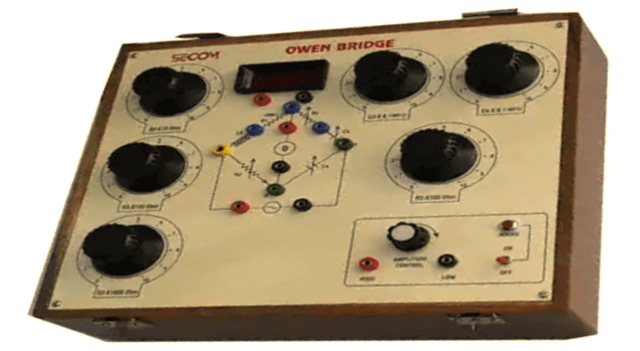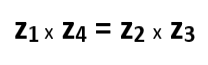Tuesday, May 30, 2023

Owen Bridge is a test equipment for measuring inductance in terms of capacitance. It works on the principle of comparison. This post will discuss what is Owen Bridge, its circuit, theory, importance, Phasor diagram of Owen’s Bridge, its advantages as well as disadvantages.

## Introduction

We have different inductor measuring bridges for measuring different quality factors. Hay’s bridge is highly suitable for measuring quality factor greater than 10. Maxwell’s bridge is highly suitable for measuring medium quality factor ranging from 1 to 10. Anderson bridge can be used successfully to measure inductor ranging from few micro Henry to several Henry.

One thing is common in all bridges i.e. they all have a limited range. We need a bridge capable of measuring a wide range of inductors. The bridge circuit that can do this is called the Owen Bridge.

## What is Owen Bridge

Owen Bridge is a test equipment for measuring inductance in terms of capacitance. It works on the comparison principle, i.e. the value of the unknown inductor is compared to the standard capacitor.Fig. 1 – Owen Bridge

Like Hay’s bridge and Maxwell Bridge, it is the AC Bridge that uses standard capacitor, inductor and variable resistors connected to the AC source for excitation.

## Owen Bridge TheoryFig. 2 – Owen Bridge Circuit Diagram

• ab, bc, cd, da are the four arms of the Owen’s Bridge. The arm ab is purely inductive and the arm bc is purely resistive in nature.
• cd has a fixed capacitor and ad has the variable resistor and capacitor connected to the circuit in series.
• The unknown inductor L1 is compared with the known capacitor C4. The bridge is kept in balance by varying R2 and C2. No current flows through the detector in balanced condition. The detector’s endpoints (b and c) have the same potential.
• Now, at the balance point, we have the AC bridge theory relationship that must hold good, i.e.Substituting the value for z1, z2, z3 we get the below equation,By separating the real and imaginary parts we get the expression for l1 and r1:Hence, the unknown inductance is calculated in this way.

## Phasor Diagram of Owen Bridge

The most lagging current is generally selected as a reference for drawing a phasor diagram.Fig. 3 – Owen Bridge Phasor Diagram

The current I2 is perpendicular to the current I1 as shown, and the drop over the inductor L1 is perpendicular to I1, as it is an inductive drop, while the drop over the capacitor C2 is perpendicular to I2. At the balance point E1 = E2, which is shown in the figure, now E1, E2, E3, E4 results from all these voltage drops.

The advantages of Owen’s Bridge include:

• The frequency does not appear in the final balance equations. So the frequency and waveform variations do not affect the bridge.
• Capacity can also be measured across a wide range, as capacity in the final balance equations is available.
• The circuit bridge of Owen is used to determine the inductance across a wide range.

The disadvantages of Owen’s Bridge include:

• The major drawback of Owen Bridge is that it uses a variable standard capacitor which is quite expensive. Also, the accuracy of this variable standard capacitor is only one percent.
• The cost increases further when measurement range of the Quality factor of coil increases. This is because the value of variable standard capacitor should also increase to measure the increased range. This further escalates the cost involved.
```Also Read:
Voltmeter – Working Principle, Voltage Sensitivity, Types and Applications
Resistance Temperature Detector, RTD – Component, Working, Application
What is Clamp Meter (Tong Tester) – Types, Operating Principle & How to Operate```Madhuri is a B.E (Computer science) and has experience in IBM as Software engineer. She is an Author, Editor and Partner at Electricalfundablog.com.
RELATED ARTICLES